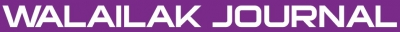### A Reliable Algorithm for Fractional Schrödinger Equations

Abid KAMRAN, Umer HAYAT, Ahmet YILDIRIM, Syed Tauseef MOHYUD-DIN

#### Abstract

In this paper, the Homotopy Perturbation Method (HPM) is applied to find exact solutions of time-fractional Schrödinger equations. Numerical results coupled with graphical representations explicitly reveal the complete reliability and efficiency of the proposed algorithm.

#### Keywords

Homotopy perturbation method, fractional Schrödinger partial differential equations, nonlinear problems, exact solution

PDF

#### References

S Abbasbandy. Numerical method for non-linear wave and diffusion equations equation by the variational iteration method. Int. J. Numer. Meth. Eng. 2008; 73, 1836-43.

S Abbasbandy. Numerical solutions of nonlinear Klein-Gordon equation by variational iteration method. Int. J. Numer. Meth. Eng. 2007; 70, 876-81.

MA Abdou and AA Soliman. New applications of variational iteration method. Physica D 2005; 211, 1-8.

A Ghorbani and JS Nadjfi. He’s homotopy perturbation method for calculating Adomian’s polynomials. Int. J. Nonlinear Sci. Num. Simul. 2007; 8, 229-332.

JH He. Some asymptotic methods for strongly nonlinear equation. Int. J. Mod. Phys. 2006; 20, 1144-99.

JH He. Some applications of nonlinear fractional differential equations and their approximations. Bull. Sci. Tech. 1999; 15, 86-90.

WX Ma and RG Zhou. Nonlinearization of spectral problems for the perturbation KdV systems. Physica A 2001; 296, 60-74.

WX Ma and M Chen. Hamiltonian and quasi-Hamiltonian structures associated with semi-direct sums of Lie algebras. J. Phys. A: Math. Gen. 2006; 39, 10787-801.

S Momani. An algorithm for solving the fractional convection-diffusion equation with nonlinear source term. Comm. Nonlinear Sci. Numer. Simulat. 2007; 12, 1283-90.

ST Mohyud-Din, MA Noor and KI Noor. Some relatively new techniques for nonlinear problems. Math. Probl. Eng. 2009; 2009, Article ID 234849.

ST Mohyud-Din and MA Noor. Homotopy perturbation method for solving fourth-order boundary value problems. Math. Probl. Eng. 2007; 2007, Article ID 98602.

ST Mohyud-Din and MA Noor. Homotopy perturbation method for solving partial differential equations. Zeitschrift für Naturforschung A 2008; 64a, 1-14.

ST Mohyud-Din. Variational iteration techniques for boundary value problems. VDM Verlag, ISBN 978-3-639-27664-0, 2010.

ST Mohyud-Din, MA Noor, KI Noor and MM Hosseini. Solution of singular equations by He’s variational iteration method. Int. J. Nonlinear Sci. Numer. Simulat. 2010; 11, 81-6.

ST Mohyud-Din, MA Noor and KI Noor. Travelling wave solutions of seventh-order generalized KdV equations using He’s polynomials. Int. J. Nonlinear Sci. Numer. Simulat. 2009; 10, 223-9.

Z Odibat and S Momani. Numerical solution of Fokker-Planck equation with space-and time-fractional derivatives. Phys. Lett. A 2007; 369, 349-58.

I Podlubny. Fractional differential equations. Academic Press, New York, 1999.

A Yildirim, H Koçak. Homotopy perturbation method for solving the space-time fractional advection-dispersion equation. Adv. Water Resour. 2009; 32, 1711-6.

A Yildirim and ST Mohyud-Din. Analytical approach to space and time fractional Burger’s equations. Chin. Phys. Lett. 2010; 27, Article ID 090501.

AM Wazwaz. The modified decomposition method and Pade´s approximants for solving Thomas-Fermi equation. Appl. Math. Comput. 1999; 105, 11-9.

L Xu. He’s homotopy perturbation method for a boundary layer equation in unbounded domain. Comput. Math. Appl. 2007; 54, 1067-70.

### Refbacks

• There are currently no refbacks.Online ISSN: 2228-835X

http://wjst.wu.ac.th

Last updated: 12 August 2019